Sequential Circuits

Sequential circuits are those with memory, also called “feedback”.  In this, they differ
from combinational circuits, which have no memory.

The stable output of a combinational circuit does not depend on the order in which its
inputs are changed.  The stable output of a sequential circuit usually does depend
on the order in which the inputs are changed.

Sequential circuits can be used as memory elements; binary values can be stored in them.
The binary value stored in a circuit element is often called that element’s state.

All sequential circuits depend on a phenomenon called gate delay.  This reflects the fact
that the output of any logic gate (implementing a Boolean function) does not change
immediately when the input changes, but only some time later.

The gate delay for modern circuits is typically a few nanoseconds.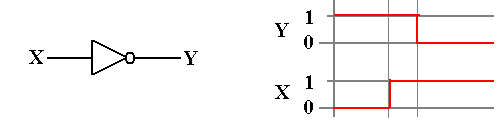Synchronous Sequential Circuits

We usually focus on clocked sequential circuits,
also called synchronous sequential circuits.

As the name “synchronous” implies, these circuits respond to a system clock,
which is used to synchronize the state changes of the various sequential circuits.

One textbook claims that “synchronous sequential circuits use clocks to order events.”  A better claim might be that the clock is used to coordinate events.  Events that should happen at the same time do; events that should happen later do happen later.

The system clock is a circuit that emits a sequence of regular pulses with a fixed and reliable pulse rate.  If you have an electronic watch (who doesn’t?), what you have is a small electronic circuit emitting pulses and a counter circuit to count them.

Clock frequencies are measured in
kilohertz                  thousands of ticks per second
megahertz               millions of ticks per second
gigahertz                 billions of ticks per second.

One can design asynchronous sequential circuits, which are not controlled by a system clock.  They present significant design challenges related to timing issues.

Views of the System Clock

There are a number of ways to view the system clock.  In general, the view depends on the detail that we need in discussing the problem.  The logical view is shown in the next figure, which illustrates some of the terms commonly used for a clock.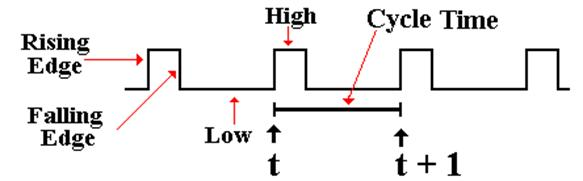The clock is typical of a periodic function.  There is a period t for which

f(t) = f(t + t)

This clock is asymmetric.  It is often the case that the clock is symmetric, where the time spent at the high level is the same as that at the low level.  Your instructor often draws the clock as asymmetric, just to show that such a design is allowed.

NOTATION:   We always call the present clock tick “t” and the next one “t + 1”, even if it occurs only two nanoseconds later.

Views of the System Clock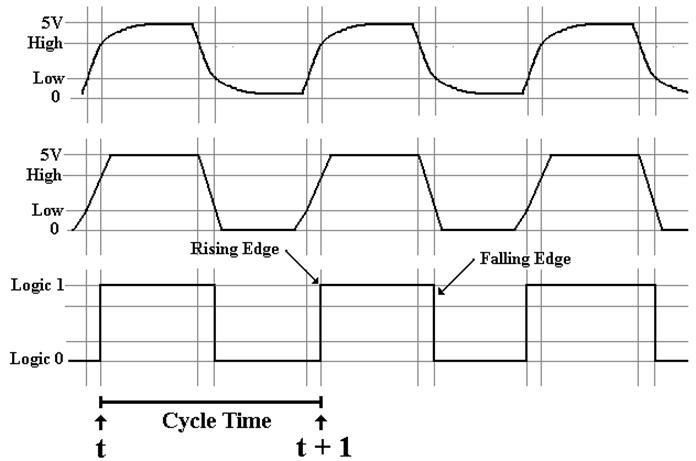The top view is the “real physical view”.  It is seldom used.
The middle view reflects the fact that voltage levels do not change instantaneously.
We use this view when considering system busses.

Clock Period and Frequency

If the clock period is denoted by t, then the frequency (by definition) is f = 1 / t.

For example, if t = 2.0 nanoseconds, also written as t = 2.0·10–9 seconds, then
f = 1 /
(2.0·10–9 seconds) = 0.50·109 seconds–1 or 500 megahertz.

If f = 2.5 Gigahertz, also written as 2.5·109 seconds–1, then
t = 1.0 / (2.5·109 seconds–1) = 0.4·10–9 seconds = 0.4 nanosecond.

Latches and Flip–Flops: First Definition

We consider a latch or a flip–flop as a device that stores a single binary value.

Flip–flops and clocked latches are devices that accept input at fixed times dictated by the system clock.  For this reason they are called “synchronous sequential circuits”.

Denote the present time by the symbol t.  Denote the clock period by t.

Rather than directly discussing the clock period, we merely say that
the current time is
t
after the next clock tick the time is (
t + 1)

The present state of the device is often called Q(t)
The next state of the device is often called Q(
t + 1)

The sequence: the present state is Q(t), the clock “ticks”, the state is now Q(t + 1)

AGAIN:          We call the next state Q(t + 1), even if the transition from Q(t) to
Q(
t + 1) takes only a few nanoseconds.  We are counting the actual
number of clock ticks, not the amount of time they take.

Latches and Flip–Flops: When Triggered

Clocked latches accept input when the system clock is at logic high.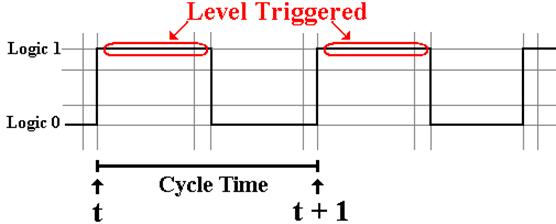Flip–flops accept input on either the rising edge of the system clock.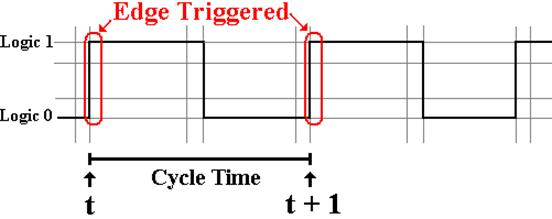When either a flip–flop or a latch is used as a part of a circuit, we have the problem of feedback.  In this, the output of the device is processed and then used as input.

Example: The flip–flop is a part of a register that is to be incremented.

We define the data path for the computer as following the output of the flip–flop through the processing elements and back to the input of the flip–flop.

The data path time is the amount of time that it takes the data to travel the data path.

If this time is too short, the processed output of the flip–flop can get back to its input during the time when the flip–flop remains sensitive to its input.

A flip–flop is a latch that has been modified to minimize the time during which the device responds to its input.

This minimizes the possibility of uncontrolled feedback as associated instabilities.

In this course, we shall ignore latches and focus only on flip–flops.

Describing Flip–Flops

A flip–flop is a “bit bucket”; it holds a single binary bit.

A flip–flop is characterized by its current state: Q(t).

We want a way to describe the operation of the flip–flops.

How do these devices respond to the input?  We use tables to describe the operation.

Characteristic tables:   Given Q(t), the present state of the flip–flop, and
the input, what will Q(
t + 1), the next state of the flip–flop, be?

Excitation tables:          Given Q(t), the present state of the flip–flop, and
Q(
t + 1), the desired next state of the flip–flop,
what input is required to achieve that change.

Functional Definition of Flip–Flops

We use the characteristic table to describe both latches and flip–flops.

The characteristic table takes the present state and input and shows the next state.

Here is the characteristic table for an SR flip–flop.

 S R Present State Next State 0 0 0 0 0 0 1 1 0 1 0 0 0 1 1 0 1 0 0 1 1 0 1 1 1 1 0 ERROR 1 1 1 ERROR

Characteristic Tables

We often take a table such as

 S R Present State Next State 0 0 0 0 0 0 1 1 0 1 0 0 0 1 1 0 1 0 0 1 1 0 1 1 1 1 0 ERROR 1 1 1 ERROR

And abbreviate it as

 S R Q(t + 1) = Next State 0 0 Q(t) 0 1 0 1 0 1 1 1 ERROR

Comment on Notation Used

All flip–flops have a number of inputs that your instructor does not indicate unless they are required for discussion of the circuit.

Power                              every flip–flop must be powered

Ground                            every flip–flop must be grounded

Clock                              all flip–flops are clocked devices

Asynchronous Clear       this allows the flip–flop to be cleared independently of the
clock.  In other words, make Q(
t) = 0.

Asynchronous Set           this allows the flip–flop to be set independently of the
clock.  In other words, make Q(
t) = 1.

Absent the explicit clock input, your instructor’s circuits might resemble unclocked latches.  Your instructor does not use such latches, but designs only with flip–flops.

SR Flip–Flop

We now adopt a functional view.  How does the next state depend on the present state and input.  A flip–flop is a “bit holder”.

Here is the diagram for the SR flip–flop.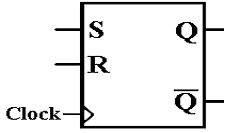Here again is the state table for the SR flip–flop.

 S R Q(t + 1) 0 0 Q(T) 0 1 0 1 0 1 1 1 ERROR

Note that setting both S = 1 and R = 1 causes the flip–flop to enter a logically inconsistent state, followed by an indeterministic, almost random, state.  For this reason, we label the output for S = 1 and R = 1 as an error.

We Need Another Flip–Flop

Consider the characteristic table for the SR flip–flop.

It is the same as that for the SR latch, except for the explicit reference to the clock.

 S R Q(t + 1) 0 0 Q(t) 0 1 0 1 0 1 1 1 ERROR

Were we to modify the SR flip–flop, what could be placed in the last row?

It is easy to see that there are only four Boolean functions of a single Boolean variable Q.
F(Q) = 0, F(Q) = Q, F(Q) =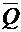, and F(Q) = 1.  The above table is missing.

This gives rise to the JK, the most general of the flip–flops.  Its characteristic table is:

 J K Q(t + 1) 0 0 Q(t) 0 1 0 1 0 1 1 1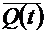JK Flip–Flop

A JK flip–flop generalizes the SR to allow for both inputs to be 1.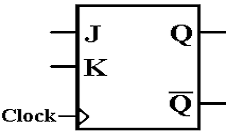Here is the characteristic table for a JK flip–flop.

 J K Q(t + 1) 0 0 Q(t) 0 1 0 1 0 1 1 1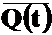Note that the flip–flop can generate all four possible functions of a single variable:
the two constants 0 and 1
the variables Q and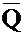.

The D Flip–Flop

The D flip–flop specializes either the SR or JK to store a single bit.  It is very useful for interfacing the CPU to external devices, where the CPU sends a brief pulse to set the value in the device and it remains set until the next CPU signal.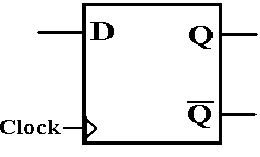The characteristic table for the D flip–flop is so simple that it is expressed better as the equation Q(t + 1) = D.  Here is the table.

 D Q(t + 1) 0 0 1 1

The T Flip–Flop

The “toggle” flip–flop allows one to change the value stored.  It is often used in circuits in which the value of the bit changes between 0 and 1, as in a modulo–4 counter in which the low–order bit goes 0, 1, 0, 1, 0, 1, etc.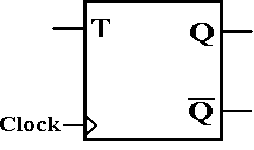The characteristic table for the D flip–flop is so simple that it is expressed better as the equation Q(t + 1) = Q(t) Å T.  Here is the table.

 T Q(t + 1) 0 Q(t) 1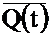Here the symbol “T” denotes the input; “t” and “t + 1” denote time.

The JK Flip–Flop as a General–Use Flip–Flop

The JK flip–flop can be used to implement any of the other three flip–flops.

As a D flip–flop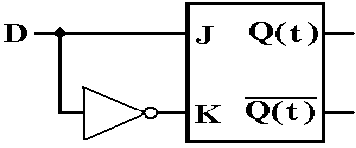As a T flip–flop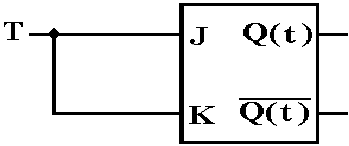As an SR flip–flop.  Just never use J = 1 and K = 1 as simultaneous inputs.

Excitation Table for an SR Flip–Flop

Here again is the state table for the SR flip–flop.

 S R Q(t + 1) 0 0 Q(t) 0 1 0 1 0 1 1 1 Error

We now derive the excitation table.

If Q(t) = 0 and we want Q(t + 1) = 0, there are two choices:
S = 0 and R = 0 maintains the same state, so Q(t + 1) = Q(t) = 0.
S = 0 and R = 1 forces Q(t + 1) = 0.
If S = 0, then the next state will be Q(t + 1) = 0 without regard to R.  We say S = 0, R = d.

Here is the Excitation Table for an SR Flip–Flop.

 Q(t) Q(t + 1) S R 0 0 0 d 0 1 1 0 1 0 0 1 1 1 d 0

JK Flip–Flop

A JK flip–flop generalizes the SR to allow for both inputs to be 1.Here is the characteristic table for a JK flip–flop.

 J K Q(t + 1) 0 0 Q(t) 0 1 0 1 0 1 1 1Here is the Excitation Table for a JK Flip–Flop.

 Q(t) Q(t + 1) J K 0 0 0 d 0 1 1 d 1 0 d 1 1 1 d 0